Courses

# Test: Heterocyclic- 1

## 20 Questions MCQ Test Organic Chemistry | Test: Heterocyclic- 1

Description
This mock test of Test: Heterocyclic- 1 for Chemistry helps you for every Chemistry entrance exam. This contains 20 Multiple Choice Questions for Chemistry Test: Heterocyclic- 1 (mcq) to study with solutions a complete question bank. The solved questions answers in this Test: Heterocyclic- 1 quiz give you a good mix of easy questions and tough questions. Chemistry students definitely take this Test: Heterocyclic- 1 exercise for a better result in the exam. You can find other Test: Heterocyclic- 1 extra questions, long questions & short questions for Chemistry on EduRev as well by searching above.
QUESTION: 1

### Pyrrole is less basic than pyridine. The orbital having the lone pair of electrons on nitrogen in pyrrole is:

Solution:

The nitrogen atom is sp2  hybridized. The N electron pair is on a p orbital, perpendicular to the plane of the ring and overlapping with the other p orbitals. There are a total of 6 π electrons, which is a Hückel number (Hückel numbers are 2, 6, 10, etc.) and, therefore,
the molecule is aromatic.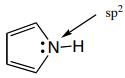QUESTION: 2

Solution:
QUESTION: 3

### Furan on prolonged heating with dimethyl acetylenedicarboxylate yields:

Solution:
QUESTION: 4

The structures of the products A and B formed in the above reaction scheme respectively are: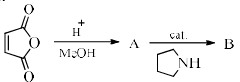Solution:
QUESTION: 5

The decreasing order of the reactivity of the following compounds towards electrophiles is: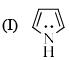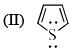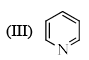Solution:
QUESTION: 6

Pyridine undergoes electrophilic nitration at elevated temperature to given the following as a major product:

Solution: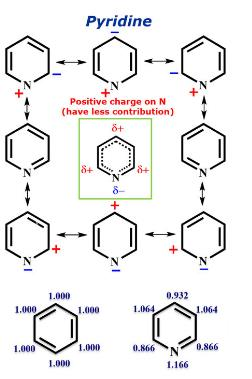There is a relatively less partial positive charge on meta positions of Pyridine. Hence, when an electrophile attacks it attacks on meta position.

Hence D is correct.

QUESTION: 7

A pyridine derivative (P) reacts with (Y). (Y) can be a free radical, cation or anion. The structure of intermediate (Q) formed in the reacts is given below. (P) and (Y) respectively are: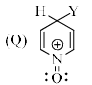Solution:
QUESTION: 8

Pyrrole + PhMgBr → E + F
E + MeCl → G + H
F + MeCl → no reaction without a catalyst.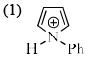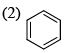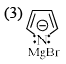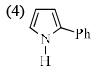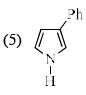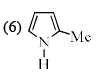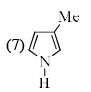The structure of products E–H, respectively are:

Solution:
QUESTION: 9

Chose the correct answer from the following four choices.

Statement: Pyridine is more basic than pyrrole.

Reason: The nitrogen in pyrrole carries a proton while the nitrogen in pyridine does not.

Assertion: Nitrogen in trigonal geometry are generally more basic than the nitrogen’s in tetrahedral geometry.

Solution:
QUESTION: 10

In the following sequence of reactions, the major product Q is: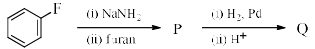Solution:
QUESTION: 11

What will be the reagent used for the completion of the following reaction?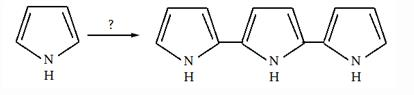Solution:
QUESTION: 12

The corrects order of the basicity of the following compound is: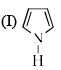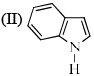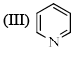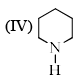Solution:
QUESTION: 13

Identify the major Product P in the following two–step reaction: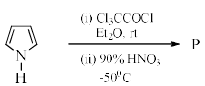Solution:
QUESTION: 14

Match the structures in List–I with their correct names in List–II.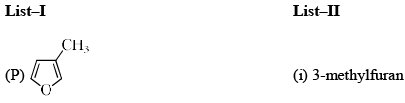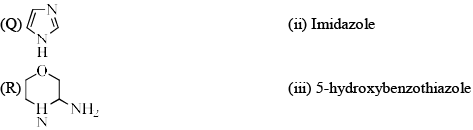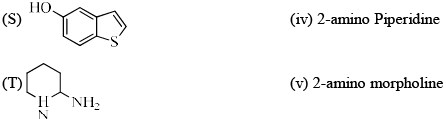Solution:
QUESTION: 15

The most acidic species is:

Solution:
QUESTION: 16

The compound that is NOT oxidized by KMnO4 is:

Solution:
QUESTION: 17

Thiophene reacts with HCHO in presence of aqueous HCl to give:

Solution:

The CH2O/HCl reagent is suitable for chloromethylation of various polycyclic aromatic hydrocarbons as well as heterocyclic aromatic compounds. Thiophene gives 2-(chloromethyl)thiophene in 40-41% isolated yield, and 4-methylimidazole gives the 5-chloromethyl derivative (51-68%).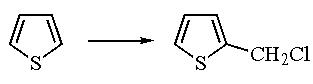QUESTION: 18

The reaction of 2-methylfuran with DMF-POCl3 would give:

Solution:
QUESTION: 19

In the following reaction the major product (X) is: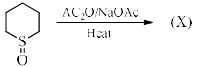Solution:
QUESTION: 20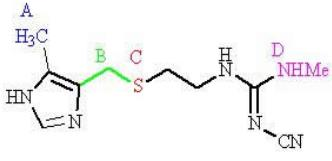Two regions of cimetidine are susceptible to metabolism. Which regions?

Solution: